# Geometrical Optics Questions and Answers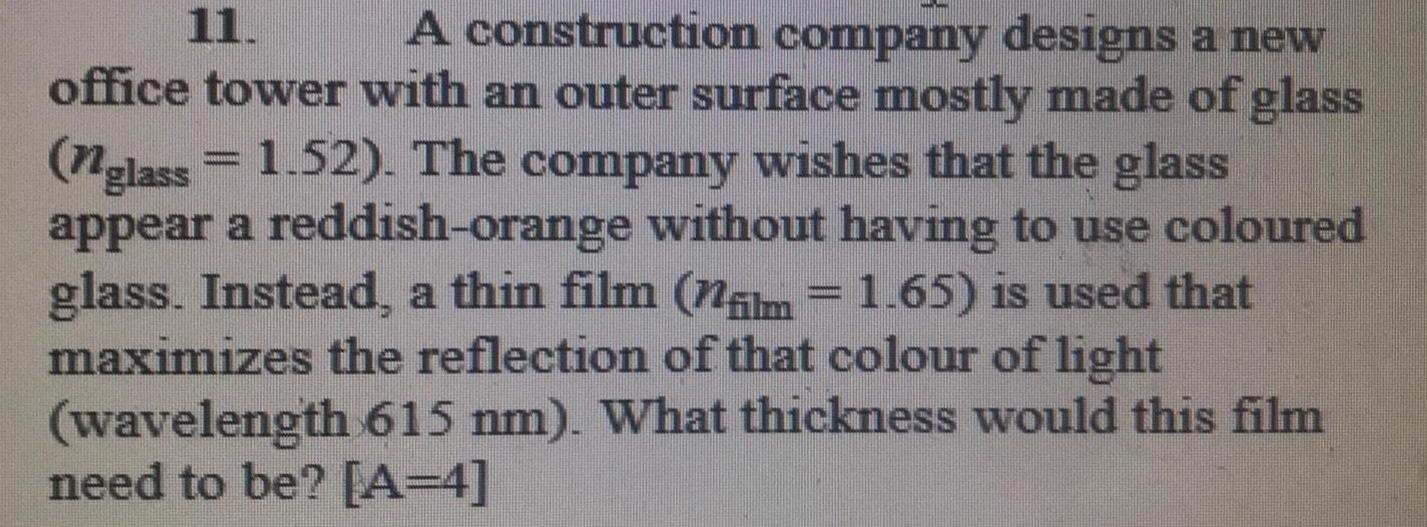Physics
Geometrical Optics
A construction company designs a new office tower with an outer surface mostly made of glass (nglass= 1.52). The company wishes that the glass appear a reddish-orange without having to use coloured glass. Instead, a thin film (film = 1.65) is used that maximizes the reflection of that colour of light (wavelength 615 nm). What thickness would this film need to be? [A=4]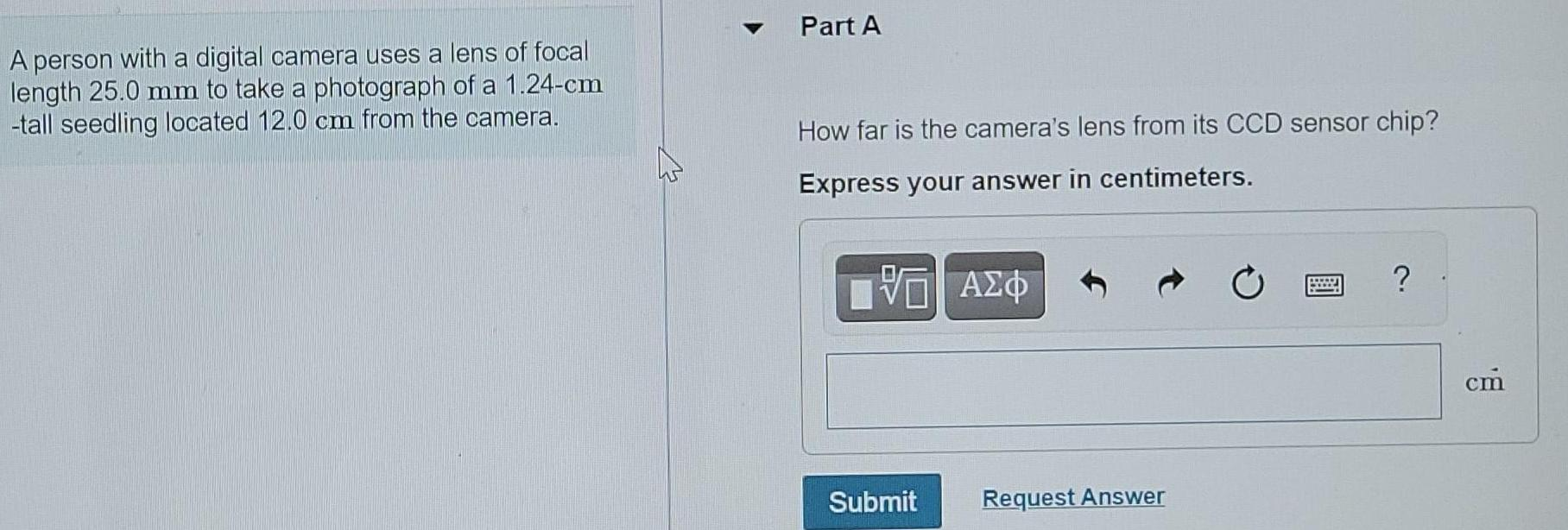Physics
Geometrical Optics
A person with a digital camera uses a lens of focal length 25.0 mm to take a photograph of a 1.24-cm -tall seedling located 12.0 cm from the camera. Part A How far is the camera's lens from its CCD sensor chip? Express your answer in centimeters.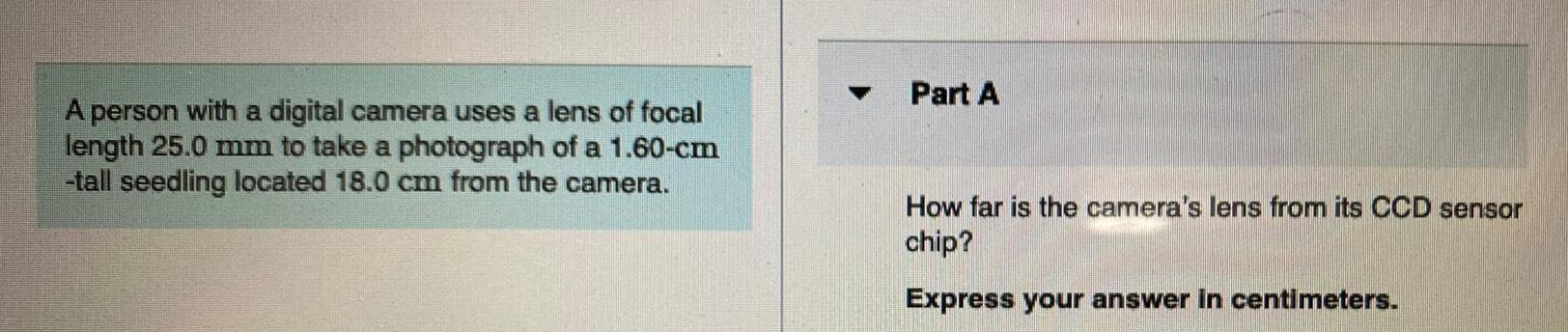Physics
Geometrical Optics
A person with a digital camera uses a lens of focal length 25.0 mm to take a photograph of a 1.60-cm -tall seedling located 18.0 cm from the camera. Part A How far is the camera's lens from its CCD sensor chip? Express your answer in centimeters.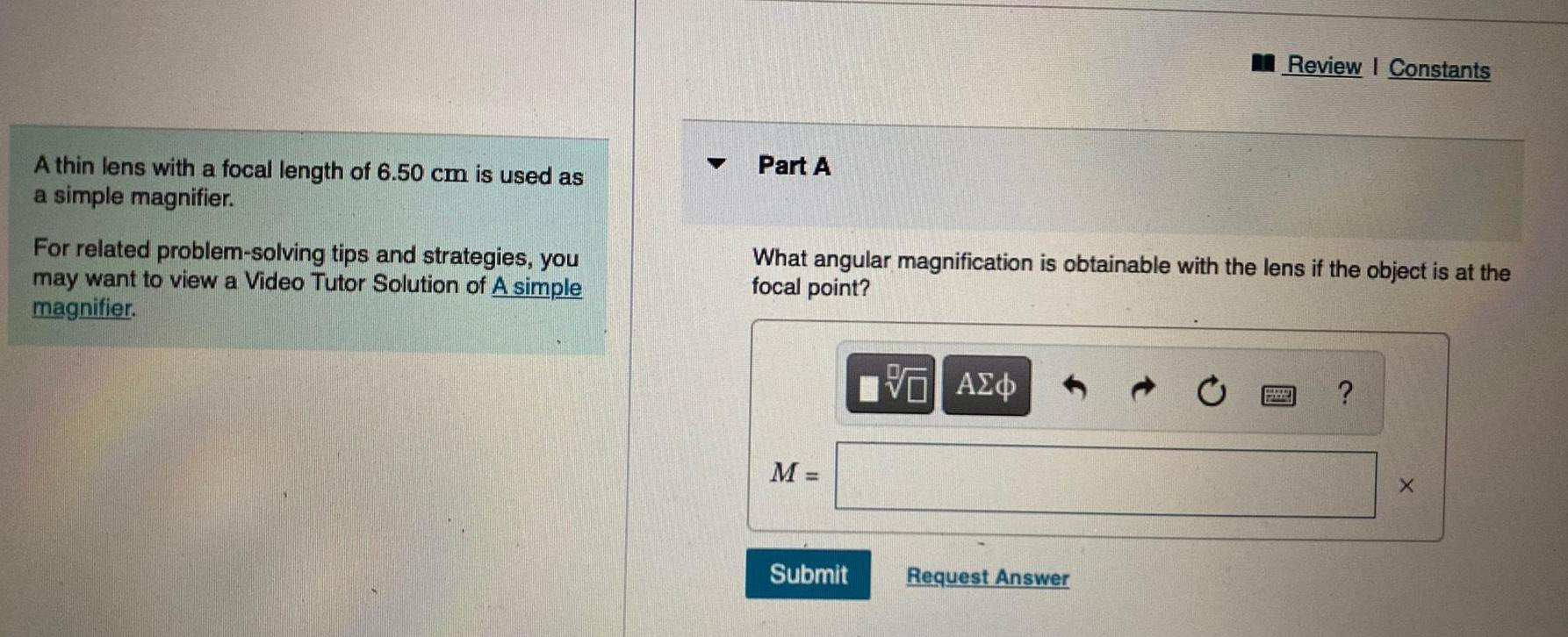Physics
Geometrical Optics
A thin lens with a focal length of 6.50 cm is used as a simple magnifier. For related problem-solving tips and strategies, you may want to view a Video Tutor Solution of A simple magnifier. Part A What angular magnification is obtainable with the lens if the object is at the focal point? M =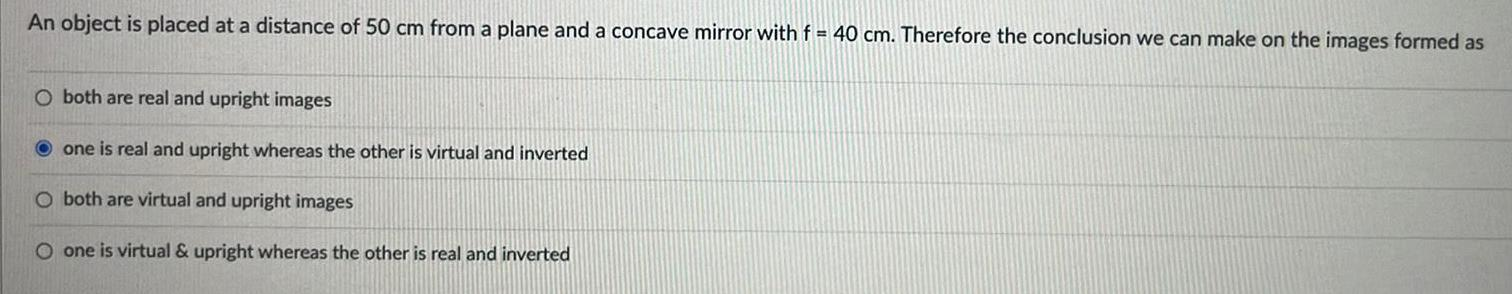Physics
Geometrical Optics
An object is placed at a distance of 50 cm from a plane and a concave mirror with f=40 cm. Therefore the conclusion we can make on the images formed as O both are real and upright images O one is real and upright whereas the other is virtual and inverted O both are virtual and upright images O one is virtual & upright whereas the other is real and inverted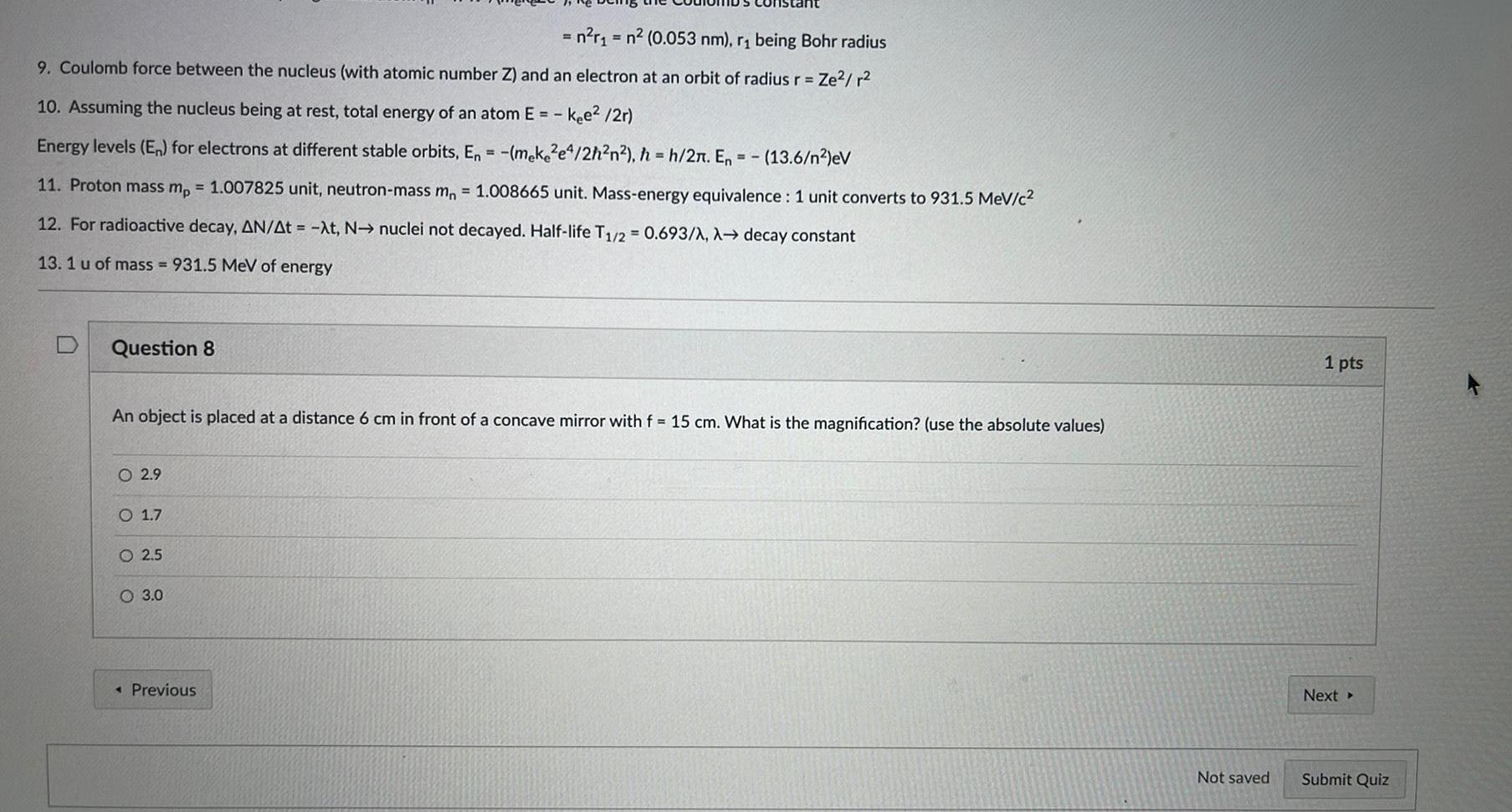Physics
Geometrical Optics
9. Coulomb force between the nucleus (with atomic number Z) and an electron at an orbit of radius r = Ze²/r² 10. Assuming the nucleus being at rest, total energy of an atom E= - kee²/2r) Energy levels (En) for electrons at different stable orbits, En = -(meke2e4/2h²n²), h=h/2π. En = -(13.6/n²)eV 11. Proton mass mp = 1.007825 unit, neutron-mass mn = 1.008665 unit. Mass-energy equivalence: 1 unit converts to 931.5 MeV/c² 12. For radioactive decay, ΔN/Δt = -λt, N→ nuclei not decayed. Half-life T1/2= 0.693/λ, λ→ decay constant 13. 1 u of mass=931.5 MeV of energy Question 8 An object is placed at a distance 6 cm in front of a concave mirror with f = 15 cm. What is the magnification? (use the absolute values) 2.9 1.7 2.5 3.0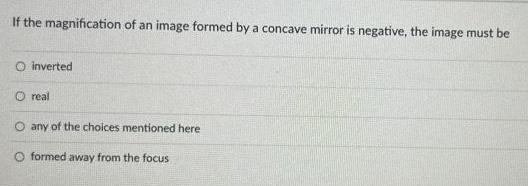Physics
Geometrical Optics
If the magnification of an image formed by a concave mirror is negative, the image must be A. inverted B. real C. any of the choices mentioned here D. formed away from the focus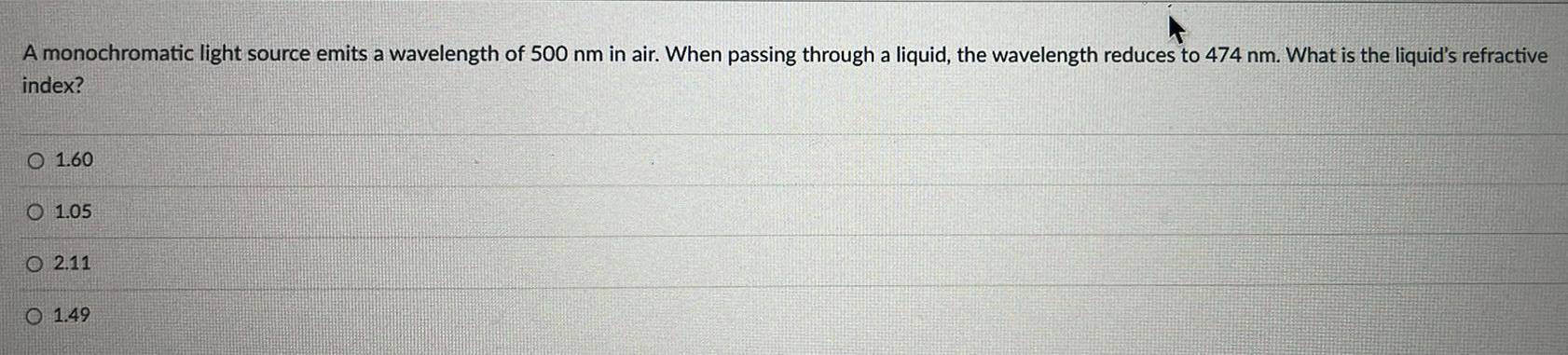Physics
Geometrical Optics
A monochromatic light source emits a wavelength of 500 nm in air. When passing through a liquid, the wavelength reduces to 474 nm. What is the liquid's refractive index? A. 1.60 B. 1.05 C. 2.11 D. 1.49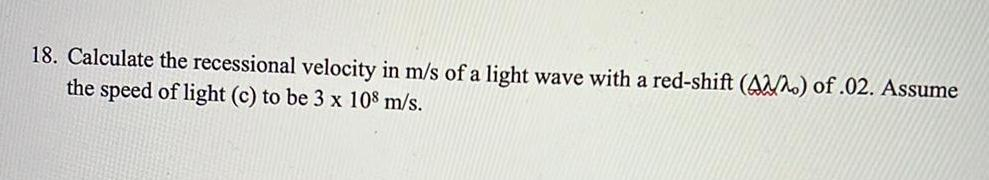Physics
Geometrical Optics
Calculate the recessional velocity in m/s of a light wave with a red-shift (Δλ/λ0) of .02. Assume the speed of light (c) to be 3x10^8 m/s.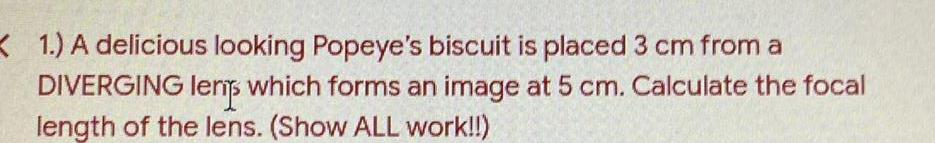Physics
Geometrical Optics
A delicious looking Popeye's biscuit is placed 3 cm from a DIVERGING lerip which forms an image at 5 cm. Calculate the focal length of the lens.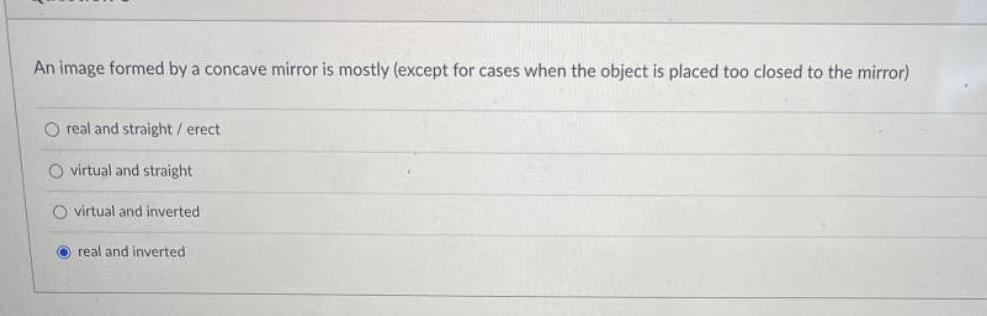Physics
Geometrical Optics
An image formed by a concave mirror is mostly (except for cases when the object is placed too closed to the mirror) real and straight / erect virtual and straight virtual and inverted real and inverted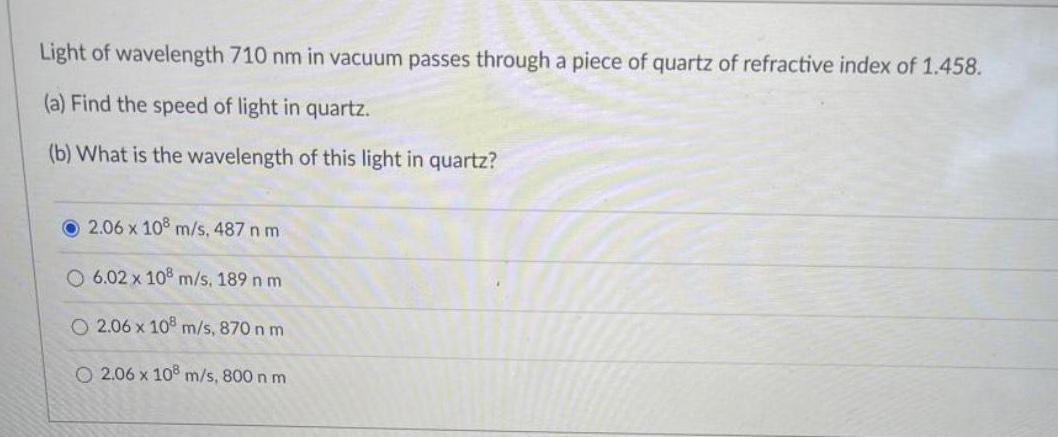Physics
Geometrical Optics
Light of wavelength 710 nm in vacuum passes through a piece of quartz of refractive index of 1.458. (a) Find the speed of light in quartz. (b) What is the wavelength of this light in quartz? O 2.06 x 108 m/s, 487 n m O 6.02 x 108 m/s, 189 n m O 2.06 x 108 m/s, 870 n m O 206 x 10® m/s,800 nm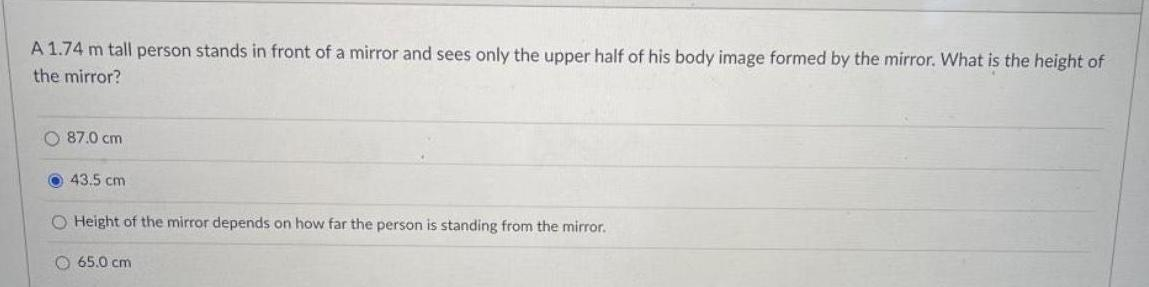Physics
Geometrical Optics
A 1.74 m tall person stands in front of a mirror and sees only the upper half of his body image formed by the mirror. What is the height of the mirror? 87.0 cm 43.5 cm Height of the mirror depends on how far the person is standing from the mirror. 65.0 cm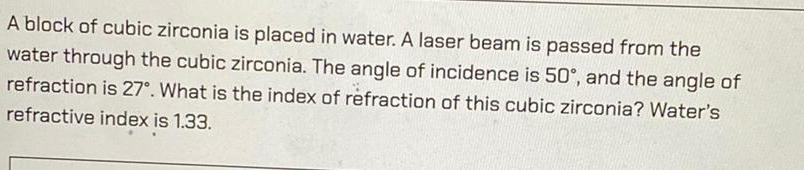Physics
Geometrical Optics
A block of cubic zirconia is placed in water. A laser beam is passed from the water through the cubic zirconia. The angle of incidence is 50°, and the angle of refraction is 27°. What is the index of refraction of this cubic zirconia? Water's refractive index is 1.33.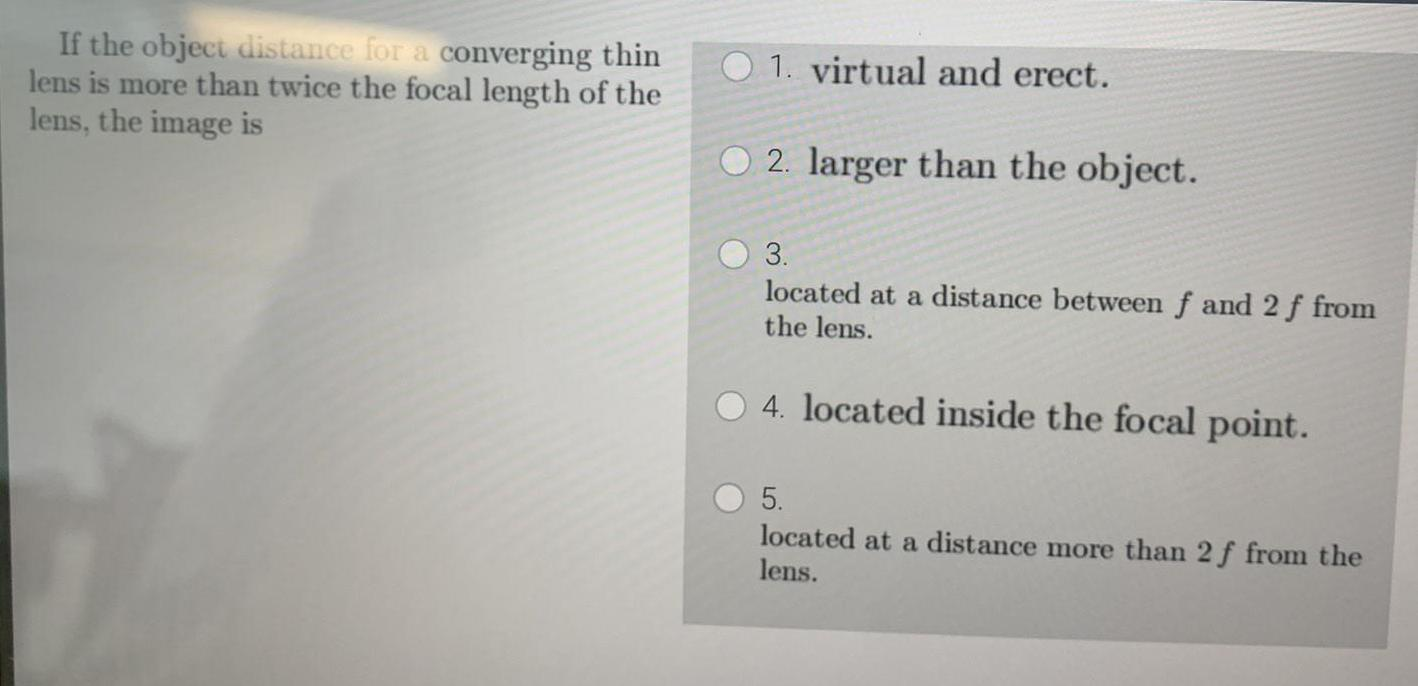Physics
Geometrical Optics
If the object distance for a converging thin lens is more than twice the focal length of the lens, the image is 1. virtual and erect. 2. larger than the object. 3. located at a distance between f and 2 f from the lens. 4. located inside the focal point. 5. located at a distance more than 2 f from the lens.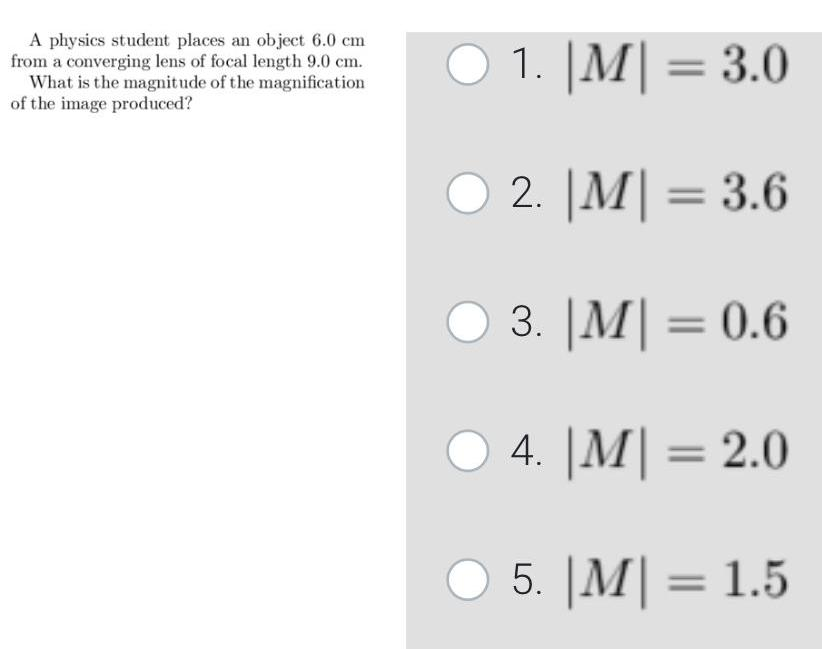Physics
Geometrical Optics
A physics student places an object 6.0 cm from a converging lens of focal length 9.0 cm. What is the magnitude of the magnification of the image produced? 1. |M| = 3.0 2. |M| = 3.6 3. |M| = 0.6 4. |M| = 2.0 5. |M| = 1.5